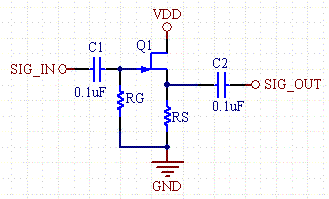# JFET Buffer Bias Calculator

A JFET buffer (Common Drain Amplifier) is useful in that it has extremely high input resistance as compared to a BJT buffer. The input resistance is equal to RG, which is typically very large, on the order of 1M ohm.Figure 1 - N-Channel JFET Buffer

 N-Channel JFET Buffer Bias VDD (V) Desired VRS (V) Vp or VGS(off) (Pinch off Voltage, negative) (V) IDSS (Zero Gate Voltage Drain Current) (mA) Results IDS (mA) RS (ohms) VGS (V)

### Equations:

IDS= IDSS(1-VGS/Vp)2

RS= VRS/IDS